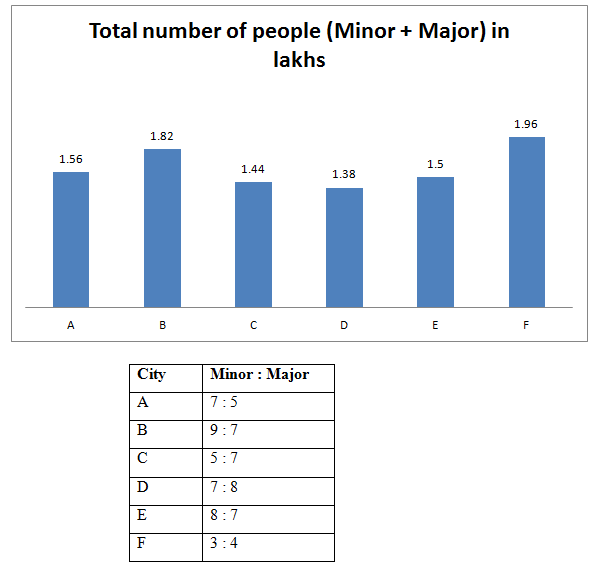# NIACL AO Prelims – Quantitative Aptitude Questions Day- 68

Dear Readers, Bank Exam Race for the Year 2019 is already started, To enrich your preparation here we have providing new series of Practice Questions on Quantitative Aptitude – Section. Candidates those who are preparing for NIACL AO Prelims 2019 Exams can practice these questions daily and make your preparation effective.

[WpProQuiz 4769]

Directions (Q. 1 – 5): What approximate value should come in place of question mark (?) in the following questions?

1) (12.78/130) of 168997 + 6.8 ÷ 13 × 272.55 + 55. 87 ÷ 8 – 12.2 × 5.1 =?

a) 18524

b) 13784

c) 16994

d) 21364

e) 25734

2) 22.87 % of 99.23 + 34.75 % of 121.23 =? – 45.12 ÷ 8.97

a) 70

b) 55

c) 85

d) 110

e) 40

3) (18.232 – 9.782) × (19.98 ÷ 160) +? % of 899 = 244

a) 13

b) 17

c) 28

d) 24

e) 32

4) (7544.89 + 5405.23 + 999.58) ÷ (2134.78 + 979.92 + 1504.68) =?

a) 8

b) 3

c) 12

d) 17

e) 23

5) 2743.78 ÷ 14 + 1716.12 ÷ 13 + 1247.99 ÷ 16 =? % of 949

a) 52

b) 36

c) 43

d) 65

e) 78

Directions (Q. 6 – 10): Study the following information carefully and answer the given questions:

The following bar graph shows the total number of people (Minor + Major) in lakhs in different cities and the table shows the ratio of minor to that of major people among them.6) Find the difference between the total number of minor people in city A and C together to that of total number of major people in city D and F together?

a) 42500

b) 36800

c) 34600

d) 40200

e) None of these

7) If the ratio between the total number of minor males to that of females in city B is 3 : 2, then find the sum of the total number of minor females in city B and the total number of major people in city E?

a) 110950

b) 132820

c) 128740

d) 95680

e) None of these

8) Find the total number of minor people in city D, E and F together?

a) 189600

b) 212800

c) 193200

d) 228400

e) None of these

9) Total number of minor people in city C is approximately what percentage of total number of major people in city D?

a) 105 %

b) 82 %

c) 73 %

d) 66 %

e) 118 %

10) Total number of major people in city B and D together is approximately what percentage more/less than the total number of major people in city A and C together?

a) 3 % less

b) 12 % less

c) 3 % more

d) 12 % more

e) 20 % less

(12.78/130) of 168997 + 6.8 ÷ 13 × 272.55 + 55. 87 ÷ 8 – 12.2 × 5.1 = x

X = (13/130)*169000 + (7*273)/13 + (56/8) – (12*5)

X = 16900 + 147 + 7 – 60 = 16994

22.87 % of 99.23 + 34.75 % of 121.23 = x – 45.12 ÷ 8.97

23 % of 100 + 35 % of 120 = x – (45/9)

23 + 42 + 5 = x

X = 70

(182 – 102) ×(20 ÷ 160) + x % of 900 = 244

(324 – 100)*(20/160) + (x/100)*900 = 244

28 + 9x = 244

9x = 216

X = 216/9 = 24

(7545 + 5405 + 1000) ÷ (2135 + 980 + 1505) = x

X = 13950/4620 = 3

2744 ÷ 14 + 1716 ÷ 13 + 1248 ÷ 16 =? % of 950

(2744/14) + (1716/13) + (1248/16) = (x/100)*950

196 + 132 + 78 = (19x/2)

X = (406*2)/19 = 43

The total number of minor people in city A and C together

= > 156000*(7/12) + 144000*(5/12)

= > 91000 + 60000 = 151000

The total number of major people in city D and F together

= > 138000*(8/15) + 196000*(4/7)

= > 73600 + 112000 = 185600

Required difference = 185600 – 151000 = 34600

The ratio between the total number of minor males to that of females in city B

= 3: 2

The total number of minor females in city B

= > 182000*(9/16)*(2/5) = 40950

The total number of major people in city E

= > 150000*(7/15) = 70000

Required sum = 40950 + 70000 = 110950

The total number of minor people in city D, E and F together

= > 138000*(7/15) + 150000*(8/15) + 196000*(3/7)

= > 64400 + 80000 + 84000 = 228400

Total number of minor people in city C

= > 144000*(5/12)

Total number of major people in city D

= > 138000*(8/15)

Required % = {[144000*(5/12)] / [138000*(8/15)]}*100 = 82 %

Total number of major people in city B and D together

= > 182000*(7/16) + 138000*(8/15)

= > 79625 + 73600 = 153225

Total number of major people in city A and C together

= > 156000*(5/12) + 144000*(7/12)

= > 65000 + 84000 = 149000

Required % = [(153225 – 149000)/149000]*100 = 3 % more